Chow variety

Chow scheme

An algebraic variety whose points parametrize all algebraic subvarietiesof dimensionand degree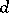of a projective space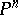.

In the product, where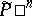is the dual of the projective space, parametrizing the hyperplanes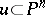, one considers the subvariety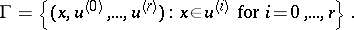Its image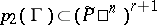under the projection onto the second factor is a hypersurface in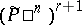which is given by a form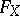in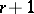systems ofvariables, homogeneous of degreein each system of variables. The formis called the associated form (or the Cayley form) of the variety. It completely determinesas a subvariety. This form was introduced by B.L. van der Waerden and W.L. Chow . The coefficients ofare determined up to a constant factor, and are called the Chow coordinates of.

The Chow coordinates of a varietydetermine a point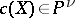, whereis a certain function ofand. The pointscorresponding to irreducible subvarietiesof dimensionand degreeform a quasi-projective subvariety, called the Chow variety. If one considers not only the irreducible subvarieties, but also positive algebraic cycles (that is, formal linear combinations of varieties with positive integer coefficients) of dimensionand degreein, then one obtains a closed subvariety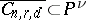, which is also called the Chow variety. The Chow variety is the base of a universal algebraic family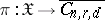, where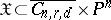,is the induced projection, and the fibre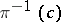above the point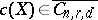is identified with the cycle. The simplest examples of Chow varieties are the varieties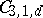of curves of degreein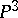. Thus,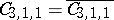is an irreducible variety of dimension 4, isomorphic to the Plücker quadric in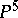;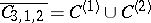consists of two components of dimension 8, where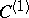corresponds to smooth curves of order two, and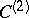to pairs of lines;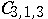consists of four components of dimension 12 corresponding to triplets of lines, curves consisting of a line together with a planar quadric, planar cubics, and non-planar curves of order 3. In all these cases the varietyis rational. However, it follows from the non-rationality of moduli schemes of curves of sufficiently high genus that for sufficiently highthe varietyis not rational (cf. ).

If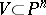is an algebraic subvariety, then the cycles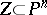of dimensionand degreethat lie inform an algebraic subvariety. This result permits one to introduce a certain algebraic structure on the set of all positive-dimensional cycleson the variety(cf. ).

For other approaches to the problem of the classification of varieties cf. Hilbert scheme; Moduli problem.